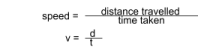# Kinematics## Kinematics

1.1: SPEED● Average speed: speed over a duration of time

● Instant speed: speed at a specific instant in time

● SI units of speed: metres per second (ms-1 or m/s)

● Speed can be measured using various lab experiments, such as light gates, ticker-timers and motion sensors

1.2: DISTANCE AND DISPLACEMENT, SCALAR AND VECTOR

● Displacement: distance moved in a particular direction (or shortest distance between starting and finishing points) (vector quantity)

● Distance: total length of the path travelled (scalar quantity).

● Consider the below example, showing the difference between displacement and distance:

If a runner completes a lap of a circular race track, what is the distance they have

covered? What is their displacement? How do the two differ?

The distance they cover is equal to the circumference of the track, since this is

the total length of the path travelled. However, their displacement would equal 0

since they finish exactly where they started (so the shortest distance between the

starting and finishing points is 0 m).

1.3: SPEED AND VELOCITY

● Speed is a scalar quantity, velocity is a vector quantity. Both are measured in ms-1. ● Velocity is the rate of change of displacement

1.4: DISPLACEMENT-TIME GRAPHS

● The different shapes of a displacement-time graph show how an object can move:● The gradient of a displacement-time graph is equal to the velocity of the object. The steeper the gradient, the larger the magnitude of the velocity

1.5: COMBINING DISPLACEMENTS

● Displacements can be combined using vector addition, to give a resultant displacement. Consider the below example:

A fighter jet flies due west for 35 km, and then flies a further 70 km at an angle of 45° north of west. Calculate its final displacement.

To solve this, we would first need to draw a vector diagram using the information given and a scale with which to make our measurements:

Then we measure the final displacement by connecting the ends of our vector diagram in the correct orientation

Finally, we multiply our measured value by the scale factor used to find the magnitude of the displacement:

Since displacement is a vector quantity, we need to consider its direction also. This is done simply by measuring the angle between the horizontal plane and the resultant displacement vector (Note: some exam questions will ask to find the angle from the vertical. Be careful when measuring the angle; make sure the correct starting point is used!). This gives the final answer:

1.6: COMBINING VELOCITIES

● In the same way that displacements can be combined, velocities can too. Consider the below example:

A boat is sailing across a river. The boat is sailing at a speed of 85 ms-1, while the river is flowing perpendicularly at a speed of 50 ms-1. Calculate the resultant velocity of the boat, giving its magnitude and angle from the vertical.

To solve this, the steps are very similar to the example above (combining displacements). We would first need to draw a vector diagram using the

information given and a scale with which to make our measurements:

Then we measure the resultant velocity by connecting the ends of our vector diagram in the correct orientation

Finally, we multiply our measured value by the scale factor used to find the magnitude of the velocity:

Since velocity is a vector quantity, we need to consider its direction also. Note that the question specifies that the angle from the vertical must be given, so it must be measured as such. This gives the final answer: